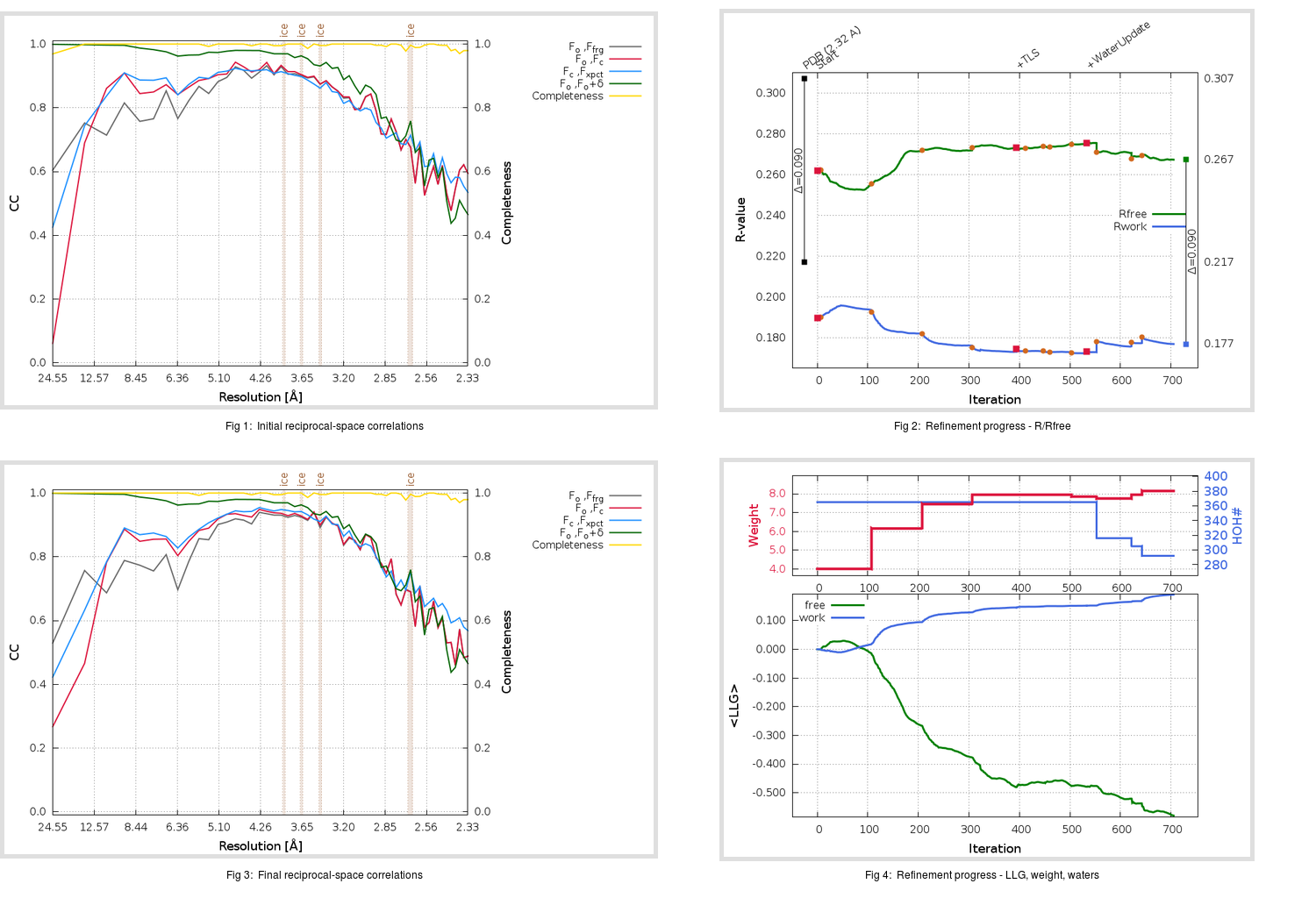Content:

## Deposited

` `
 Date deposited Date data collection Resolution R, Rfree 20200315 20200305 2.32 0.2120 0.3070

Molprobity (CCP4 7.0 version) summary:

```Ramachandran outliers =   0.33 %
favored =  95.36 %
Rotamer outliers      =   2.56 %
C-beta deviations     =     0
Clashscore            =   3.07
RMS(bonds)            =   0.0130
RMS(angles)           =   1.71
MolProbity score      =   1.73
Resolution            =   2.32
R-work                =   0.2120
R-free                =   0.3070
```

```Number of waters      =   365

<B> (all atoms) =   33.08 ( sd =   12.27 ) for       2834 non-hydrogen atoms
<B>   (protein) =   33.33 ( sd =   12.04 ) for       2440 non-hydrogen atoms
<B>     (water) =   30.31 ( sd =   13.00 ) for        365 non-hydrogen atoms
<B>    (others) =   42.45 ( sd =   18.39 ) for         29 non-hydrogen atoms

B min/max       (all non-hydrogen atoms) =    0.50 /  105.84
B min/max   (protein non-hydrogen atoms) =   16.15 /  105.84
B min/max     (water non-hydrogen atoms) =    0.50 /   82.52
B min/max     (other non-hydrogen atoms) =   26.54 /   96.47
```

## BUSTER (re-)refinement

` `

Molprobity (CCP4 7.0 version) summary:

```Ramachandran outliers =   0.00 %
favored =  96.36 %
Rotamer outliers      =   2.93 %
C-beta deviations     =     0
Clashscore            =   7.17
RMS(bonds)            =   0.0119
RMS(angles)           =   1.61
MolProbity score      =   1.99
Resolution            =   2.32
R-work                =   0.1769
R-free                =   0.2673
```

```Number of waters      =   292

<B> (all atoms) =   34.25 ( sd =    9.67 ) for       2761 non-hydrogen atoms
<B>   (protein) =   34.43 ( sd =    8.88 ) for       2440 non-hydrogen atoms
<B>     (water) =   31.97 ( sd =   14.58 ) for        292 non-hydrogen atoms
<B>    (others) =   39.87 ( sd =   18.68 ) for         29 non-hydrogen atoms

B min/max       (all non-hydrogen atoms) =    3.00 /   74.19
B min/max   (protein non-hydrogen atoms) =   19.26 /   74.19
B min/max     (water non-hydrogen atoms) =    3.00 /   72.28
B min/max     (other non-hydrogen atoms) =   30.02 /   64.02
```

Refinement progression:Results:

` `
 File Remark 5RFG_aB_refine.01_03_refine.pdb.gz exact refinement commands are in header 5RFG_aB_refine.01_03_refine.mtz.gz including original deposited data and several re-refinement map coefficients 5RFG_aB_refine.01_03_BUSTER_model.cif.gz including any non-standard compound restraints 5RFG_aB_refine.01_03_BUSTER_refln.cif.gz